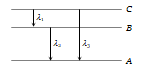An electron has a mass of . It revolves round the nucleus in a circular orbit of radius $0.529×{10}^{-10}$ metre at a speed of . The magnitude of its linear momentum in this motion is

(a) kg-m/s             (b) $2.0×{10}^{-24}$ kg-m/s
(c) $4.0×{10}^{-24}$ kg-m/s           (d) $4.0×{10}^{-31}$ kg-m/s

Concept Questions :-

Various atomic model
High Yielding Test Series + Question Bank - NEET 2020

Difficulty Level:

The ionization potential for second He electron is
(a) 13.6 eV                 (b) 27.2 eV
(c) 54.4 eV                 (d) 100 eV

Concept Questions :-

Bohr's model of atom
High Yielding Test Series + Question Bank - NEET 2020

Difficulty Level:

The energy required to remove an electron in a hydrogen atom from n = 10 state is
(a) 13.6 eV                   (b) 1.36 eV
(c) 0.136 eV                  (d) 0.0136 eV

Concept Questions :-

Bohr's model of atom
High Yielding Test Series + Question Bank - NEET 2020

Difficulty Level:

Every series of hydrogen spectrum has an upper and lower limit in wavelength. The spectral series which has an upper limit of wavelength equal to 18752 Å is
(a) Balmer series                (b) Lyman series
(c) Paschen series               (d) Pfund series

(Rydberg constant R = $1.097×{10}^{7}$ per metre)

Concept Questions :-

Spectral series
High Yielding Test Series + Question Bank - NEET 2020

Difficulty Level:

The kinetic energy of the electron in an orbit of radius r in hydrogen atom is (e = electronic charge)
(a) $\frac{{\mathrm{e}}^{2}}{{\mathrm{r}}^{2}}$                (b) $\frac{{\mathrm{e}}^{2}}{2\mathrm{r}}$
(c) $\frac{{\mathrm{e}}^{2}}{\mathrm{r}}$                (d) $\frac{{\mathrm{e}}^{2}}{2{\mathrm{r}}^{2}}$

Concept Questions :-

Bohr's model of atom
High Yielding Test Series + Question Bank - NEET 2020

Difficulty Level:

Energy levels A, B, C of a certain atom corresponding to increasing values of energy i.e. ${\mathrm{E}}_{\mathrm{A}}<{\mathrm{E}}_{\mathrm{B}}<{\mathrm{E}}_{\mathrm{C}}$. If  are the wavelengths of radiations corresponding to the transitions C to B, B to A and C to A respectively, which of the following statements is correct(a) ${\mathrm{\lambda }}_{3}={\mathrm{\lambda }}_{1}+{\mathrm{\lambda }}_{2}$                      (b) ${\mathrm{\lambda }}_{3}=\frac{{\mathrm{\lambda }}_{1}{\mathrm{\lambda }}_{2}}{{\mathrm{\lambda }}_{1}+{\mathrm{\lambda }}_{2}}$
(c) ${\mathrm{\lambda }}_{1}+{\mathrm{\lambda }}_{2}+{\mathrm{\lambda }}_{3}=0$                 (d) ${\mathrm{\lambda }}_{3}^{2}={\mathrm{\lambda }}_{1}^{2}+{\mathrm{\lambda }}_{2}^{2}$

Concept Questions :-

Spectral series
High Yielding Test Series + Question Bank - NEET 2020

Difficulty Level:

The angular momentum of electron in nth orbit is given by

(a) nh                 (b) $\frac{\mathrm{h}}{2\mathrm{\pi n}}$
(c) $\mathrm{n}\frac{\mathrm{h}}{2\mathrm{\pi }}$             (d) ${\mathrm{n}}^{2}\frac{\mathrm{h}}{2\mathrm{\pi }}$

Concept Questions :-

Bohr's model of atom
High Yielding Test Series + Question Bank - NEET 2020

Difficulty Level:

The ratio of the energies of the hydrogen atom in its first to second excited state is
(a) 1/ 4                    (b) 4/9
(c) 9/ 4                     (d) 4

Concept Questions :-

Bohr's model of atom
High Yielding Test Series + Question Bank - NEET 2020

Difficulty Level:

An electron jumps from the 4th orbit to the 2nd orbit of hydrogen atom. Given the Rydberg's constant R = . The frequency in Hz of the emitted radiation will be
(a) $\frac{3}{16}×{10}^{5}$           (b) $\frac{3}{16}×{10}^{15}$
(c) $\frac{9}{16}×{10}^{15}$          (d) $\frac{3}{4}×{10}^{15}$

Concept Questions :-

Bohr's model of atom
High Yielding Test Series + Question Bank - NEET 2020

Difficulty Level:

The ionisation potential of hydrogen atom is 13.6 volt. The energy required to remove an electron in the n = 2 state of the hydrogen atom is
(a) 27.2 eV                (b) 13.6 eV
(c) 6.8 eV                  (d) 3.4 eV

Concept Questions :-

Bohr's model of atom